Q#22, S#4, SAT Test#3

This one is tricky! Here’s why: they talk about three numbers, but they really only give you enough information to write two equations, and they only ask for the value of one of the three numbers. So it looks way more complicated than it really is.

Let’s come up with some equations. First, the sum of three numbers is 855. Easy enough:And then the second thing they tell you: x is 50% more than the sum of the other two numbers.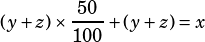That simplifies to (or maybe you prefer shorthand and just started by writing this) the following:Now we can solve by substituting or eliminating. I think substitution is a little easier here since we already have an x = equation: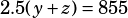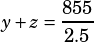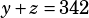Now that you’ve got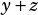, all you need to do is solve for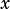.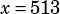(Note that, while I didn’t do this in my explanation because I think it might have been confusing, you can also just use a single letter (like a) instead of yz to represent the sum of the other two numbers, since we never need to split them apart.)